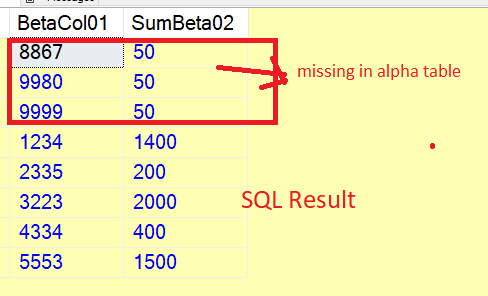# Comparing 2 table's values to see how many of them are common

Hey guys,
I'm looking for a solution to this problem.

I have 2 tables: ALPHA and BETA.
In ALPHA: I have a column called "AlphaCol01"
In BETA: I have 2 columns called "BetaCol01" and "BetaCol02"

So it's the following format with these values (image attached below):
TABLE: ALPHA
Column: AlphaCol01
Values: 1234, 1256, 1278, 2335, 2994, 3112, 3223, 3445, 3667, 4334, 4667, 4889, 5553, 5567, 5598

TABLE: BETA
Column: BetaCol01
Values: 1234, 2335, 3223, 4334, 5553, 1234, 1234, 3223, 3223, 5553, 8867, 9980, 9999

Column2: BetaCol02
Values: 100, 200, 300, 400, 500, 600, 700, 800, 900, 1000, 1100, 1200, 0

And what I'd like to get, is:

1. If we're joining BETA table's "BetaCol01" column to ALPHA table's "AlphaCol01", I'd like to get a list of values grouped by "BetaCol02" where the list contains all the values, summed and then grouped by BetaCol02, where the value of BetaCol02 can be found in ALPHA table's AlphaCol01 column.
So this result, basically:

2. I'd like to get a number (in percentage) to see that how many distinct BetaCol01 values can be found in AlphaCol01 column. So in the original example, we have 8 distinct values in BetaCol01, and out of those 8, 4 of them doesn't have any matches for AlphaCol01, so the result is 50%.

``````DROP TABLE IF EXISTS #ALPHA, #BETA
CREATE TABLE #ALPHA(AlphaCol01 int NOT NULL)
CREATE TABLE #BETA(BetaCol01 int NOT NULL,BetaCol02 int NOT NULL)

INSERT #ALPHA VALUES
(1234),(1256),(1278),(2335),(2994),(3112),(3223),(3445),(3667)
,(4334),(4667),(4889),(5553),(5567),(5598)

INSERT #BETA VALUES
(1234,100),(2335,200),(3223,300),(4334,400),(5553,500),(1234,600),(1234,700)
,(3223,800),(3223,900),(5553,1000),(8867,1100),(9980,1200),(9999,0)

SELECT b.BetaCol01, SUM(b.BetaCol02) SumBeta02
FROM #BETA b
INNER JOIN #ALPHA a ON b.BetaCol01=a.AlphaCol01
GROUP BY b.BetaCol01

;WITH beta_count(b) AS (SELECT COUNT(DISTINCT BetaCol01) FROM #BETA)
,missing(a) AS (SELECT COUNT(*) FROM #BETA b
WHERE NOT EXISTS(SELECT 1 FROM #ALPHA a WHERE b.BetaCol01=a.AlphaCol01))
SELECT b, a, a*\$100/b percentage FROM beta_count CROSS JOIN missing
``````

I'm using \$100 as a money datatype to allow the rounding to decimal places, as math on integers will truncate decimals.

Thank you, much appreciated!

hi

another way of doing this
.. a couple of things need to be clarified = percentage
.. how do you want to do the percentage ( the sum which you divide by does it include mssing from alpa table

``````SELECT
b.BetaCol01
,  case when a.AlphaCol01 IS NULL THEN 50 else SUM(b.BetaCol02)  end SumBeta02
FROM
#BETA b
LEFT JOIN
#ALPHA a ON b.BetaCol01=a.AlphaCol01
GROUP BY
b.BetaCol01,a.AlphaCol01
``````